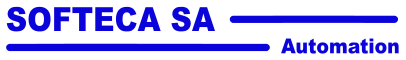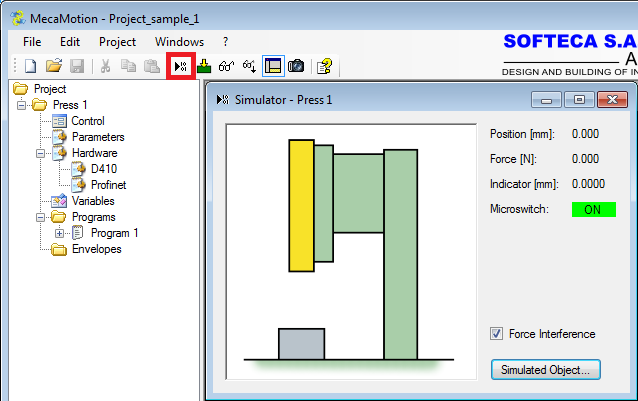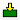# Presse universelle MecaMotion parNavigation: Programming the press with MecaMotion Simulator

Simulator

The simulator allows you to test all the functions that can be used in a part-program without needing a real press.

It allows to simulate the movement of the press, the different forces involved and visualize the results obtained.

Access the simulator

The simulator can be accessed from the main menu by clicking on the icon circled in red in the figure below. (figure 1)Figure 1: Simulator opening

The simulator works exactly like the real press. Once you have created the programs you want to test, you must load them into the simulator using thebutton . Then you can start program cycles and view the results from the "control" window.

Simulator configuration

If you want to make the force/position curve more realistic, you can check the "Force interferences" box (Figure 1) to simulate the noise found on the actual force sensor signal.

In the simulator window (figure 1), if you click on the "simulated object" button you can define according to the position of the axis which force is applied on it, this allows you to simulate a force/position curve. (figure2)

Currently the monitoring of envelopes is not simulated, exceeding the tolerance will not result in the programmed reaction.Figure 2: Simulation parameters